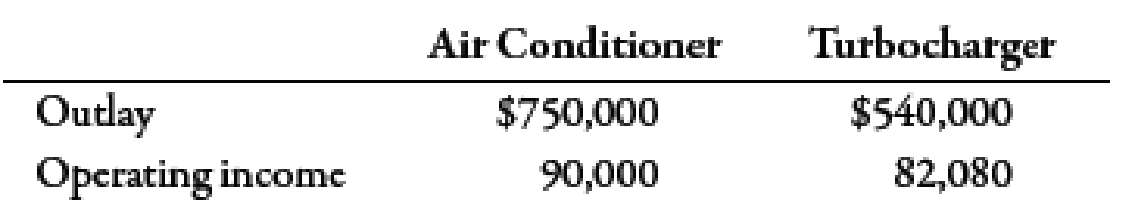Chapter 11, Problem 42P### Managerial Accounting: The Corners...

7th Edition
Maryanne M. Mowen + 2 others
ISBN: 9781337115773

#### Solutions

Chapter
Section### Managerial Accounting: The Corners...

7th Edition
Maryanne M. Mowen + 2 others
ISBN: 9781337115773
Textbook Problem
868 views

# Return on Investment for Multiple Investments, Residual IncomeThe manager of a division that produces add-on products for the automobile industry has just been presented the opportunity to invest in two independent projects. The first is an air conditioner for the back seats of vans and minivans. The second is a turbocharger. Without the investments, the division will have average assets for the coming year of $28.9 million and expected operating income of$4.335 million. The outlay required for each investment and the expected operating incomes are as follows:(Note: Round all numbers to two decimal places.)Required: 1. Compute the ROI for each investment project. 2. Compute the budgeted divisional ROI for each of the following four alternatives: a. The air conditioner investment is made. b. The turbocharger investment is made. c. Both investments are made. d. Neither additional investment is made. 3. CONCEPTUAL CONNECTION Assuming that divisional managers are evaluated and rewarded on the basis of ROI performance, which alternative do you think the divisional manager will choose? 4. CONCEPTUAL CONNECTION Suppose that the company sets a minimum required rate of return equal to 14%. Calculate the residual income for each of the following four alternatives: a. The air conditioner investment is made. b. The turbocharger investment is made. c. Both investments are made. d. Neither additional investment is made. Which option will the manager choose based on residual income? Explain. 5. CONCEPTUAL CONNECTION Suppose that the company sets a minimum required rate of return equal to 10%. Calculate the residual income for each of the following four alternatives: a. The air conditioner investment is made. b. The turbocharger investment is made. c. Both investments are made. d. Neither additional investment is made. Based on residual income, are the investments profitable? Why does your answer differ from your answer in Requirement 3?

1.

To determine

Calculate ROI for each investment project.

Explanation

Return on Investment (ROI):

Return on investment can be defined as the amount of profit earned by the company on per dollar of investment. It can be computed by dividing operating income by the average operating assets.

Use the following formula to calculate ROI for Air conditioner:

ROI=Operating IncomeAverage operating assets

Substitute $90,000 for operating income and$750,000 for average operating assets in the above formula.

ROI=$90,000$750,000=0

2.

To determine

Calculate the budgeted divisional ROI for each alternative.

3.

To determine

Identify the appropriate alternative that a divisional manager should select.

4.

To determine

Calculate residual income for each alternative with a 14% rate of return.

5.

To determine

Calculate residual income for each alternative with a 10% rate of return.

### Still sussing out bartleby?

Check out a sample textbook solution.

See a sample solution

#### The Solution to Your Study Problems

Bartleby provides explanations to thousands of textbook problems written by our experts, many with advanced degrees!

Get Started